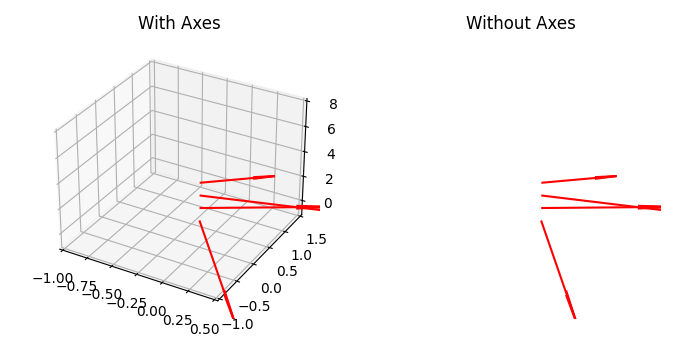# How can I hide the axes in Matplotlib 3D?

To hide the axes in matplotlib 3D, we can take the following steps−

• Create a 2D array, where x, y, z, u, v and w are the coordinates of the arrow locations and direction components of the arrow vectors.
• Using figure() method, create a new figure or activate an existing figure.
• Add an '~.axes.Axes' to the figure as part of a subplot arrangement, using add_subplot() method
• Plot a 3D field of arrows, using quiver() method.
• Using ylim, xlim, zlim, limit the range of the axes
• Set the title of the plot.
• Create two axes (ax1 and ax2). Set the titles "With Axes" and "Without Axes". Using set_axis_off() method, we can hide the axes.
• To display the figure, use show() method.

## Example

import matplotlib.pyplot as plt
import numpy as np
plt.rcParams["figure.figsize"] = [7.00, 3.50]
plt.rcParams["figure.autolayout"] = True
soa = np.array([[0, 0, 1, 1, -2, 0], [0, 0, 2, 1, 1, 0],
[0, 0, 3, 2, 1, 0], [0, 0, 4, 0.5, 0.7, 0]])
X, Y, Z, U, V, W = zip(*soa)
fig = plt.figure()
ax.quiver(X, Y, Z, U, V, W, color='red')
ax.set_xlim([-1, 0.5])
ax.set_ylim([-1, 1.5])
ax.set_zlim([-1, 8])
ax.set_title("With Axes")
ax1.set_axis_off()
ax1.quiver(X, Y, Z, U, V, W, color='red')
ax1.set_xlim([-1, 0.5])
ax1.set_ylim([-1, 1.5])
ax1.set_zlim([-1, 8])
ax1.set_title("Without Axes")
plt.show()

## Output